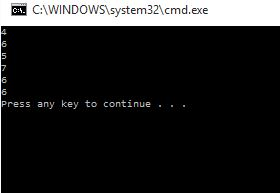# Increment and decrement operators in C#

##### How to perform Increment and Decrement operation in c#?

Increment and Decrement operator:

Increment and decrement operators are used to increase and decrease the values. (++) operator is used to increase the values ,The increment operator (++) increments its operand by 1. ( -- ) operator is used to decrease the values. The ( -- ) operator decrements its operands by -1.

```using System;
using System.Collections.Generic;
using System.Linq;
using System.Text;

class Program
{
static void Main()
{
int a = 4, b = 5;
Console.WriteLine(a++);
Console.WriteLine(++b);
Console.WriteLine(a++);
Console.WriteLine(++b);

Console.WriteLine(a--);
Console.WriteLine(--b);
}
}
```

In the above code snippet we have declared increment and decrement operators we have taken a and b values as 4 and 5.

In the first line we are declaring (a++) a value as 4 which prints the output as 4 and  a value is incremented by 1 then a value becomes 5.

In the second line we have declared (++b) the b value is 5 we are incrementing the b value with 1 it becomes 6.

In the third line we are declaring (a++), it prints the a value as 5 and it increments with 1 and the a value becomes 6.

In the fourth line (++b) we are incrementing the b value with 1 the b value is printed as 7.

In the fifth line we declaring (a--), it prints the value as 6 and the a value is decremented by 1, a value becomes 5.

In the sixth line we are declaring (--b), we are decrementing the b value by 1, the  b value prints as 6.

In the seventh line we are performing the (a--), a value is 5 and the a value is decremented by 1 then the a value becomes 4.

In the last line we are performing (--b), the b value is decremented by 1 then the b value is decremented from 6 to 5 and prints the value b as 5.

output:Views: 7114 | Post Order: 9